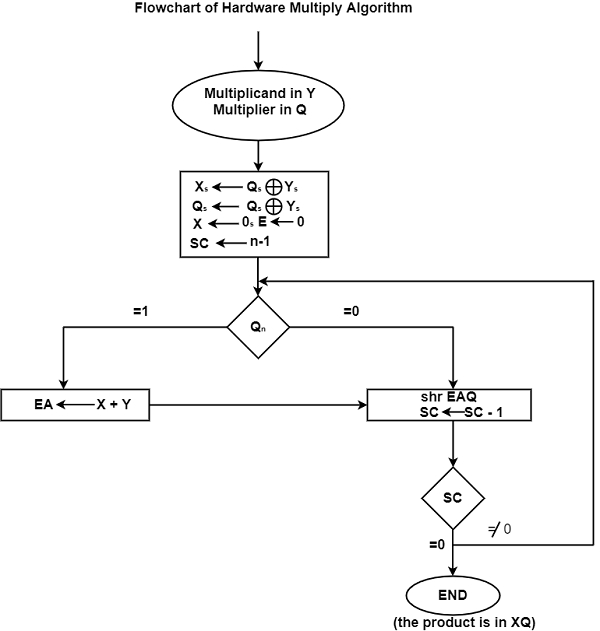# Discuss the Hardware Algorithm in Computer Architecture?

An algorithm to multiply two numbers is known as the multiplication algorithm. The hardware multiply algorithm is used in digital electronics such as computers to multiply binary digits. The figure shows the flowchart for the hardware multiply algorithm.In the flowchart shown in the figure, the multiplicand is in Y and the multiplier is in Q. The signs related to Y8 and Q8 are in respectively. These signs are compared and both X and Q are set to correspond to the sign of the product because a double-length product will be stored in registers X and Q.

The registers X and E are cleared. Then, the Sequence Counter (SC) is set to a number that is similar to the several bits of the multiplier.

It is assumed that the operands are transferred from a memory unit to the registers having words of n bits. One bit of the word is occupied by the sign and the magnitude comprises n - 1 bits because the operand has to be stored with its sign.

Once the initialization is done, the low-order bit of multiplier in is tested. In case the bit is 1, the multiplicand in Y is inserted to the present partial product that is saved in X. In case the bit is 0, no action is performed.

The SC is decreased by 1 and its new value is checked. In case it is not equal to 0, the process is repeated and a new partial product is formed. This process is halted when SC is equal to 0.

The partial product that is generated in X is shifted to Q, one bit at a time, and replaces the multiplier eventually. The final product is saved in X and Q. Here, X contains the MSBs and Q contains the Least Significant Bits (LSBs).